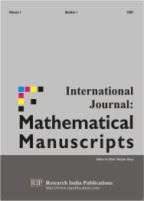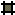International Journal: Mathematical Manuscripts (IJMM)

ISSN  0974- 2883

Editor-in-chief:Prof. Haydar Akça,

Abu Dhabi University

College of Arts and Science

Department of Applied Sciences and Mathematics

P.O Box 59911, Abu Dhabi, USE

E-mail: hakca@uaeu.ac.ae

Aims and Scope: The International Journal: Mathematical Manuscripts (IJMM) is an international research journal, which publishes top-level original and review papers, in ordinary and partial differential equations with an interest at the intersection of analysis and applications. Areas and subareas of interest include (but are not limited to) classical topics of the theory of differential equations, applied mathematics and different kinds of nonlocal interactions, dynamical systems, Combinatorics; Graph theory; ODE, PDE; Functional equations; Integral and differential inequalities and inclusions; Differential games; Control theory; Dynamical systems; Probability, statistics and stochastic processes; Statistical mechanics; Quantum theory; Relativity and gravitational theory; Integral and differential operators; Fractional calculus; Special functions; Generalized functions; Operator research and algebraic analysis; Fluid mechanics; Mechanics of particles and systems; Mathematical programming and optimization; Approximation theory; Numerical algebraic or transcendental equations; Numerical analysis for ordinary differential equations; Numerical analysis for partial differential equations; Numerical methods in complex analysis; Numerical methods in linear algebra; Numerical simulation; Acceleration of convergence; Interval arithmetic; Information systems; Software engineering; Software technology; System theory; Theory of data; Programming and image processing; Pattern recognition; Parallel and distributed algorithms; Communication systems; Computer aided design; Computer arithmetic and numerical analysis; Computer aspects of numerical algorithms; Computer networks; Concurrent and parallel computations; Data base; Discrete mathematics in relation to computer science; Manufacturing systems; Applications in mechanics, physics, chemistry, biology, technology, economics and industrial problems..

Publication Date and Frequency: Two issues per year.

Annual Subscription Price:

Library/ Institutional: Print : US\$320.00 Online Only: US\$300.00

Print + Online : US\$ 360.00

Individual/ Personnel: Print US\$160.00Vol. 3  No.1&2Vol. 4  No.1Vol. 5  No.1Editorial BoardAuthors InformationSubscriptionCall for PapersOther JournalsLibrary Recommendation Form

 © All rights are reserved with Research India Publications                             Home  Journals  Contact